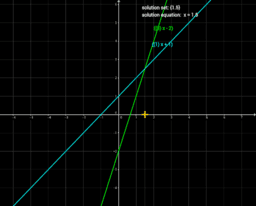# Equations & Inequalities as Comparisons of Functions

This is a series of applets drawn from the website mathMINDhabits [sites.google.com/site/mathmindhabits] that is designed for teachers of mathematics who want to deepen their understanding of the mathematics they teach and that their students are expected to learn. There are two common uses of the equal sign in mathematics, comparison and assignment. When we speak of comparisons of functions we are dealing, in general, with two different functions. [In special cases, such as identities, we may be dealing with two different symbolic forms of the same function.] Equations, inequalities, relations and identities are all examples of comparisons of functions. On the other hand, when we speak of assignments we are dealing with the problem of giving a name to a single function. Formulas and definitions are examples of assigning a name to a single function. In short, assignments deal with one function – comparisons deal with two functions. This distinction is reflected in the symbolic manipulation problems we ask students to work on. Problems of the form “factor”, “expand”, “simplify” “collect terms”, etc. are all problems that require the student to rewrite a single function in an equivalent symbolic form. Problems of the form “solve” require the student to work on (one or more) comparison(s) of functions. Equations and relations, which are written with an equal sign between two functions are comparisons of functions. When we write the equation 5x = x + 12 the equal sign does not signify that the function 5x is the same as the function x + 12. It really should be interpreted as an equal sign with a question mark overlaid. The equation 5x = x + 12 should be interpreted to mean “for what value or values of x does the function to the left of the = yield the same value or values as the function to the right?” On the other hand, the equal sign that appears in the statement F(x) = x + 12 has a different meaning – it means that F(x) is the name we assign to the function x + 12. Inequalities behave in a similar fashion. Inequalities are also comparisons of functions. The inequality 5x < x + 12 should be interpreted to mean “for what values of x does the function to the left of the < sign, i.e., 5x, yield values that are smaller than the values yielded by the function to the right of the < sign, i.e. x + 12.?